# Transgression

(diff) ← Older revision | Latest revision (diff) | Newer revision → (diff)

in a fibre space

A certain special correspondence between the cohomology classes of the fibre and the base. More precisely, ifis a connected fibre space with baseand fibreandis an Abelian group, then a transgression inis the correspondencedefined by the formulawhere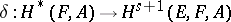is the coboundary homomorphism and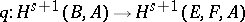is the homomorphism determined by the projection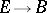. The elements of the domain of definitionof the correspondenceare called transgressive; any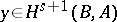such that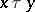is called the image of the element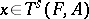under transgression. A transgression can be regarded as a homomorphism of the group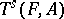into some quotient group of. The transgression has a transparent interpretation in terms of the spectral sequence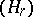of the fibre space: in essence, it is the same as the differential.

The description of transgressive cohomology classes of the fibre is very important in the study of the cohomological structure of fibre bundles. An important role is played here by the Borel transgression theorem: Ifis a field,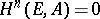for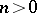,is the exterior algebra over a subspace, graded in odd degrees, where the cohomology spaces of the fibres form a simple sheaf over, thencan be chosen such that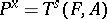for any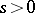; furthermore,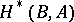is the algebra of polynomials in images of elements of a homogeneous basis ofunder the transgression. In particular, ifis a connected Lie group without-torsion and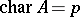, then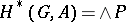, where the homogeneous elements ofhave odd degree and are transgressive in any principal fibre bundle of the group. Herecoincides with the space of primitive cohomology classes.

How to Cite This Entry:
Transgression. Encyclopedia of Mathematics. URL: http://encyclopediaofmath.org/index.php?title=Transgression&oldid=17798
This article was adapted from an original article by A.L. Onishchik (originator), which appeared in Encyclopedia of Mathematics - ISBN 1402006098. See original article